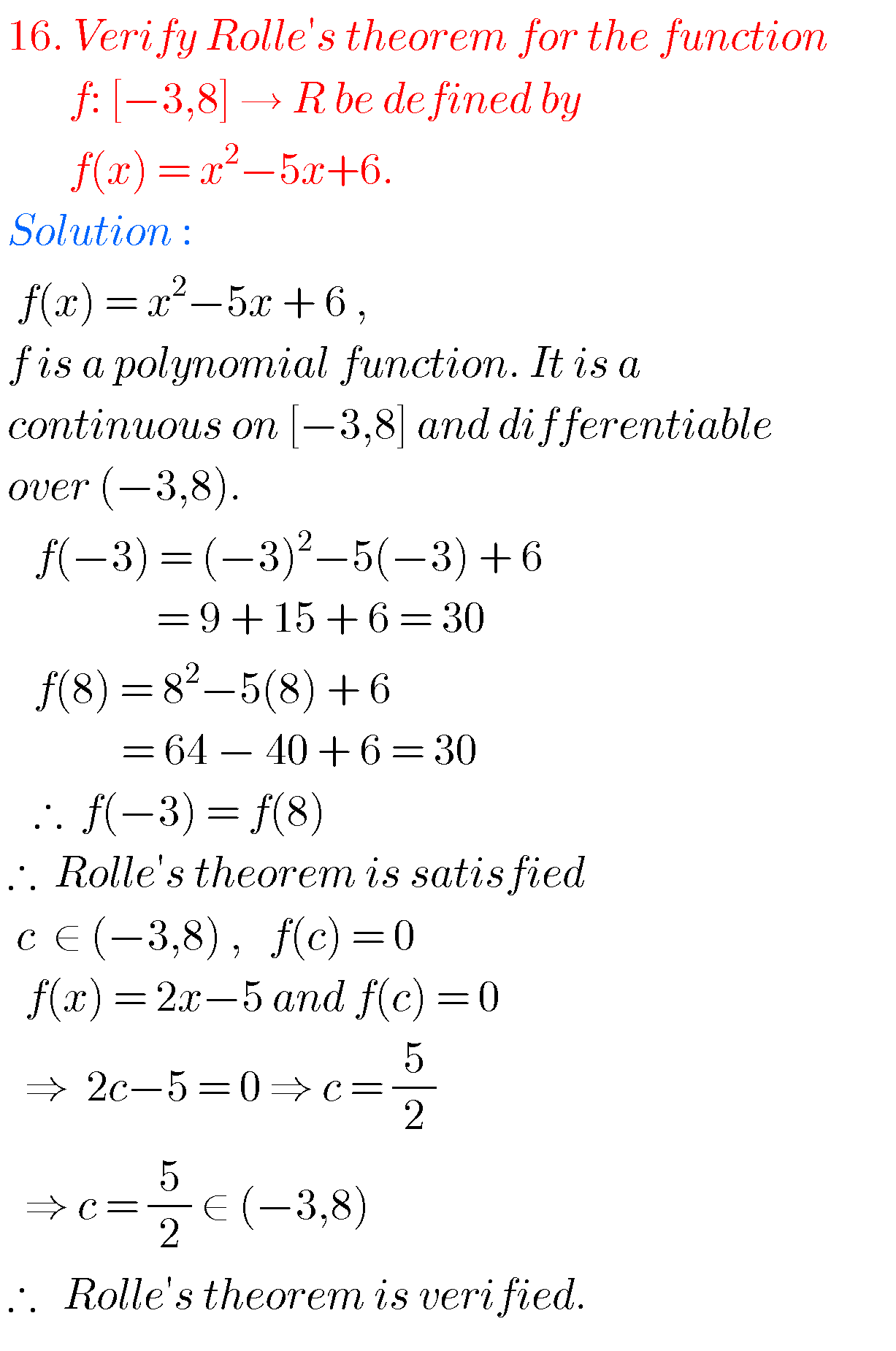## Mean Value Theorems,Intermediate first year Mathematics chapter 10.4 problems with solutions

Mean Value theorems, Intermediate first year Mathematics problems with solutions Mathematics intermediate first year 1B solutions for some problems.  These solutions are very simple to understand.  Study the textbook lesson Applications of Derivatives very well  Textbook exercise solutions links are also given. Applications of Derivatives Exercise 10(a) Exercise 10(b) Exercise 10(c) Exercise 10(d) Exercise 10(e) Exercise …